Home
Hostname: page-component-cf9d5c678-cnwzk Total loading time: 0.263 Render date: 2021-08-01T05:57:09.238Z Has data issue: true Feature Flags: { "shouldUseShareProductTool": true, "shouldUseHypothesis": true, "isUnsiloEnabled": true, "metricsAbstractViews": false, "figures": true, "newCiteModal": false, "newCitedByModal": true, "newEcommerce": true, "newUsageEvents": true }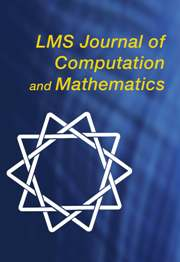LMS Journal of Computation and Mathematics

# Modular elliptic curves over the field of twelfth roots of unity

Published online by Cambridge University Press:  01 April 2016

Corresponding

## Abstract

In this paper we perform an extensive study of the spaces of automorphic forms for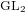$\text{GL}_{2}$ of weight$2$ and level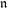$\mathfrak{n}$ , for$\mathfrak{n}$ an ideal in the ring of integers of the quartic CM field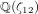$\mathbb{Q}({\it\zeta}_{12})$ of twelfth roots of unity. This study is conducted through the computation of the Hecke module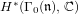$H^{\ast }({\rm\Gamma}_{0}(\mathfrak{n}),\mathbb{C})$ , and the corresponding Hecke action. Combining this Hecke data with the Faltings–Serre method for proving equivalence of Galois representations, we are able to provide the first known examples of modular elliptic curves over this field.

Type
Research Article
Information

## References

Ash, A., Gunnells, P. E. and McConnell, M., ‘Resolutions of the Steinberg module for GL(n)’, J. Algebra 349 (2012) 380390.CrossRefGoogle Scholar
Ash, A., Gunnells, P. E. and McConnell, M., ‘Mod 2 homology of GL(4) and Galois representations’, J. Number Theory 146 (2015) 422.CrossRefGoogle Scholar
Berger, T. and Harcos, G., ‘ -adic representations associated to modular forms over imaginary quadratic fields’, Int. Math. Res. Not. IMRN 23 (2007) Art. ID rnm113.Google Scholar
Blasius, D. and Rogawski, J., ‘Galois representations for Hilbert modular forms’, Bull. Amer. Math. Soc. (N.S.) 21 (1989) no. 1, 6569.CrossRefGoogle Scholar
Borel, A. and Serre, J. P., ‘Corners and arithmetic groups’, Comment. Math. Helv. 48 (1973) 436491; with an appendix, ‘Arrondissement des variétés à coins’, by A. Douady and L. Hérault.CrossRefGoogle Scholar
Bump, D., Automorphic forms and representations , Cambridge Studies in Advanced Mathematics 55 (Cambridge University Press, Cambridge, 1998).Google Scholar
Carayol, H., ‘Sur les représentations -adiques associées aux formes modulaires de Hilbert’, Ann. Sci. Éc. Norm. Supér. (4) 19 (1986) 409468.CrossRefGoogle Scholar
Carayol, H., ‘Modular forms and Galois representations with values in a complete local ring’, Contemp. Math. 165 (1994) 213237.CrossRefGoogle Scholar
Chênevert, G., ‘Exponential sums, hypersurfaces with many symmetries and Galois representations’, PhD Thesis, McGill University, Montreal, 2008.Google Scholar
Computational Algebra Group, University of Sydney, ‘MAGMA, Version 2.20-9’, 2014, http://magma.maths.usyd.edu.au/magma.Google Scholar
Donnelly, S., Gunnells, P. E., Klages-Mundt, A. and Yasaki, D., ‘A table of elliptic curves over the cubic field of discriminant − 23’, Exp. Math. 24 (2015) no. 4, 375390.CrossRefGoogle Scholar
Dieulefait, L. V., Guerberoff, L. and Pacetti, A., ‘Proving modularity for a given elliptic curve over an imaginary quadratic field’, Math. Comp. 79 (2010) 11451170.CrossRefGoogle Scholar
Freitas, N., Le Hung, B. and Siksek, S., ‘Elliptic curves over real quadratic fields are modular’, Invent. Math. 201 (2015) 159206.CrossRefGoogle Scholar
Gunnells, P. E., Hajir, F. and Yasaki, D., ‘Modular forms and elliptic curves over the field of fifth roots of unity’, Exp Math. 22 (2013) no. 2, 203216.CrossRefGoogle Scholar
Gunnells, P. E., ‘Computing Hecke eigenvalues below the cohomological dimension’, Exp. Math. 9 (2000) no. 3, 351367.CrossRefGoogle Scholar
Gunnells, P. E., ‘Lectures on computing cohomology of arithmetic groups’, Computations with modular forms , Contributions in Mathematical and Computational Sciences 6 (Springer, Cham, 2014) 345.CrossRefGoogle Scholar
Gunnells, P. E. and Yasaki, D., ‘Modular forms and elliptic curves over the cubic field of discriminant 23’, Int. J. Number Theory 9 (2013) no. 1, 5376.CrossRefGoogle Scholar
Harder, G., ‘Eisenstein cohomology of arithmetic groups. The case GL2 ’, Invent. Math. 89 (1987) 37118.CrossRefGoogle Scholar
Harder, G., ‘Cohomology of arithmetic groups’, 2006,http://www.math.uni-bonn.de/people/harder/Manuscripts/.Google Scholar
Hida, H., Elementary theory of L-functions and Eisenstein series , London Mathematical Society Student Texts 26 (Cambridge University Press, Cambridge, 1993).CrossRefGoogle Scholar
Harris, M., Lan, K. W., Taylor, R. and Thorne, J., ‘On the rigid cohomology of certain Shimura varieties’, Preprint, 2013, http://www.math.ias.edu/∼rtaylor/rigcoh.pdf.Google Scholar
Harris, M., Soudry, D. and Taylor, R., ‘ -adic representations associated to modular forms over imaginary quadratic fields. I. Lifting to GSp4(ℚ)’, Invent. Math. 112 (1993) no. 2, 377411.CrossRefGoogle Scholar
Jarvis, F. and Manoharmayum, J., ‘On the modularity of supersingular elliptic curves over certain totally real number fields’, J. Number Theory 128 (2008) no. 3, 589618.CrossRefGoogle Scholar
Jones, A., ‘Modular elliptic curves over quartic CM fields’, PhD Thesis, University of Sheffield, 2015,http://tberger.staff.shef.ac.uk/Jones_thesis.pdf.Google Scholar
Koecher, M., ‘Beiträge zu einer Reduktionstheorie in Positivitätsbereichen. I’, Math. Ann. 141 (1960) 384432.CrossRefGoogle Scholar
Mok, C. P., ‘Galois representations attached to automorphic forms on GL2 over CM fields’, Compos. Math. 150 (2014) no. 4, 523567.CrossRefGoogle Scholar
Scholze, P., ‘On torsion in the cohomology of locally symmetric varieties’, Ann. of Math. (2) 182 (2015) no. 3, 9451066.CrossRefGoogle Scholar
Schwermer, J., ‘The cohomological approach to cuspidal automorphic representations’, Cont. Math. 488 (2006) 257285.CrossRefGoogle Scholar
Stein, W., Modular forms, a computational approach , Graduate Studies in Mathematics 79 (American Mathematical Society, Providence, RI, 2007), with an appendix by Paul E. Gunnells.CrossRefGoogle Scholar
Taylor, R., ‘On Galois representations associated to Hilbert modular forms’, Invent. Math. 98 (1989) 265280.CrossRefGoogle Scholar
Taylor, R., ‘ -adic representations associated to modular forms over imaginary quadratic fields. II’, Invent. Math. 116 (1994) 619643.CrossRefGoogle Scholar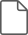### Jones supplemtary material

PDF of Tabular Data

File 261 KBYou have Access
2
Cited by

# Send article to Kindle

Note you can select to send to either the @free.kindle.com or @kindle.com variations. ‘@free.kindle.com’ emails are free but can only be sent to your device when it is connected to wi-fi. ‘@kindle.com’ emails can be delivered even when you are not connected to wi-fi, but note that service fees apply.

Find out more about the Kindle Personal Document Service.

Modular elliptic curves over the field of twelfth roots of unity
Available formats
×

# Send article to Dropbox

To send this article to your Dropbox account, please select one or more formats and confirm that you agree to abide by our usage policies. If this is the first time you use this feature, you will be asked to authorise Cambridge Core to connect with your <service> account. Find out more about sending content to Dropbox.

Modular elliptic curves over the field of twelfth roots of unity
Available formats
×

# Send article to Google Drive

To send this article to your Google Drive account, please select one or more formats and confirm that you agree to abide by our usage policies. If this is the first time you use this feature, you will be asked to authorise Cambridge Core to connect with your <service> account. Find out more about sending content to Google Drive.

Modular elliptic curves over the field of twelfth roots of unity
Available formats
×
×